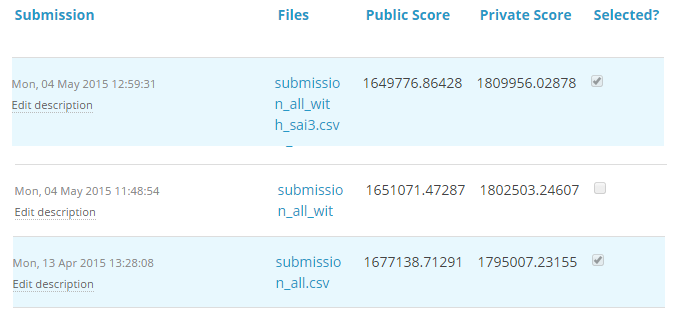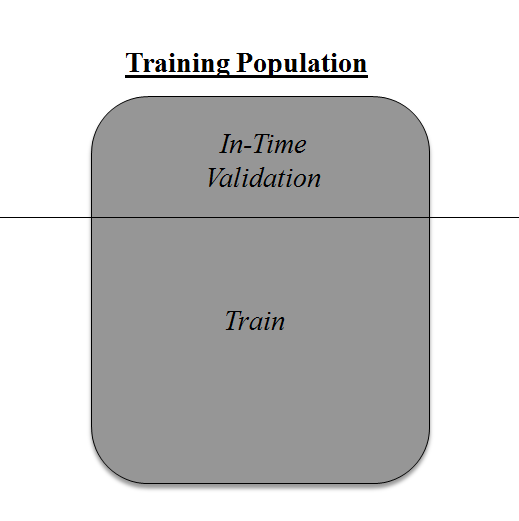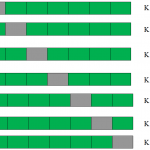# Cross Validation in Detail

## Cross Validation

Let’s first understand the importance of cross validation. Due to busy schedules, these days I don’t get much time to participate in data science competitions. Long time back, I participated in TFI Competition on Kaggle. Without delving into my competition performance, I would like to show you the dissimilarity between my public and private leaderboard score.

### Here is an example of scoring on Kaggle!

For TFI competition, following were three of my solution and scores (Lesser the better) :You will notice that the third entry which has the worst Public score turned to be the best model on Private ranking. There were more than 20 models above the “submission_all.csv”, but I still chose “submission_all.csv” as my final entry (which really worked out well). What caused this phenomenon ? The dissimilarity in my public and private leaderboard is caused by over-fitting.

Over-fitting is nothing but when you model become highly complex that it starts capturing noise also. This ‘noise’ adds no value to model, but only inaccuracy.

In the following section, I will discuss how you can know if a solution is an over-fit or not before we actually know the test results.

### The concept : Cross Validation

Cross Validation is one of the most important concepts in any type of data modelling. It simply says, try to leave a sample on which you do not train the model and test the model on this sample before finalizing the model.Above diagram shows how to validate model with in-time sample. We simply divide the population into 2 samples, and build model on one sample. Rest of the population is used for in-time validation.

Could there be a negative side of the above approach?

I believe, a negative side of this approach is that we loose a good amount of data from training the model. Hence, the model is very high bias. And this won’t give best estimate for the coefficients. So what’s the next best option?

What if, we make a 50:50 split of training population and the train on first 50 and validate on rest 50. Then, we train on the other 50, test on first 50. This way we train the model on the entire population, however on 50% in one go. This reduces bias because of sample selection to some extent but gives a smaller sample to train the model on. This approach is known as 2-fold cross validation.

### k-fold Cross validation

Let’s extrapolate the last example to k-fold from 2-fold cross validation. Now, we will try to visualize how does a k-fold validation work.This is a 7-fold cross validation.

Here’s what goes on behind the scene : we divide the entire population into 7 equal samples. Now we train models on 6 samples (Green boxes) and validate on 1 sample (grey box). Then, at the second iteration we train the model with a different sample held as validation. In 7 iterations, we have basically built model on each sample and held each of them as validation. This is a way to reduce the selection bias and reduce the variance in prediction power. Once we have all the 7 models, we take average of the error terms to find which of the models is best.